# Example of configuring an Excel template in APFill software to display the page size in inches or millimeters

Starting from APFill 5.6, the program allows users to use MS Excel files as templates for processing the results of document toner coverage measurements.

If the default template is used, APFill will process a PDF file and generate a new Excel file of the following form containing the results of CMYK toner coverage measurements.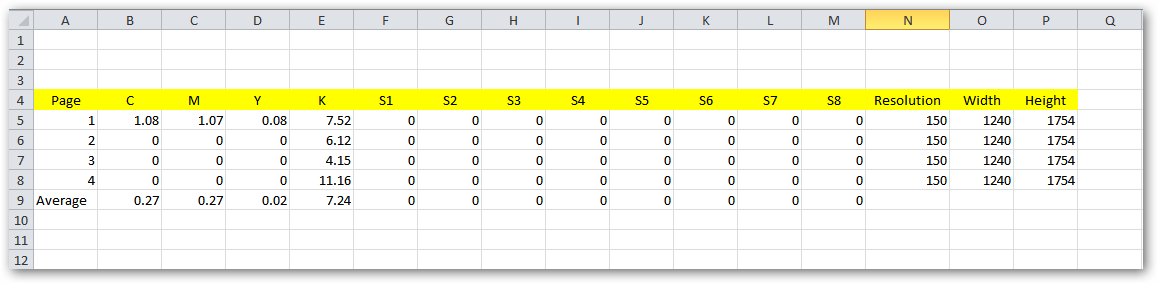Let’s consider a situation where we need to specify the size of each page in inches or millimeters. APFill shows the coverage ratio for each page in the results table, as well as its size in pixels and the resolution used for PDF file rasterization. This data is sufficient for determining the size of each page in inches or millimeters. To do it, you need to add extra columns containing the formulas below to the Excel template.

`Page Width in inches = Page Width in pixels/Resolution`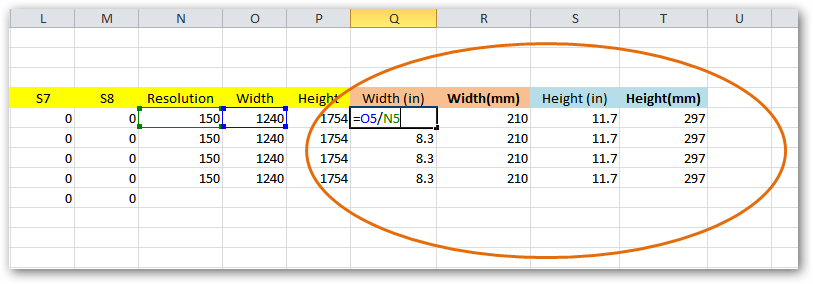`Page Width in millimeters = (Page Width in pixels/Resolution)*25.4`
Where 25.4 is the ratio for converting inches to millimeters.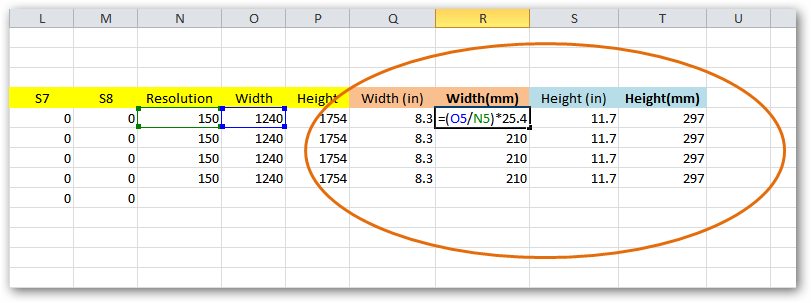Identical formulas are added for the page height.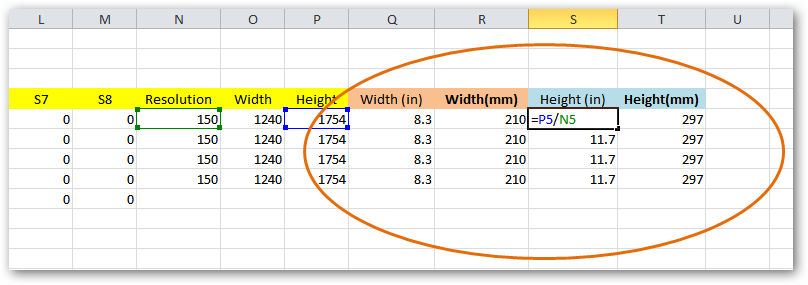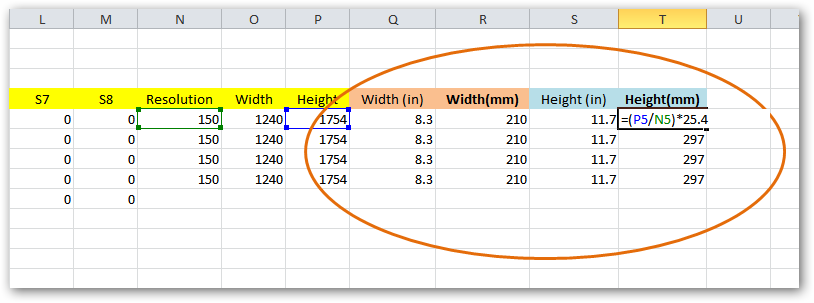This is how adding formulas to the default template helps you configure APFill to calculate all the necessary CMYK toner coverage measurements for a PDF file.